# Numerical Simulation of the Characteristics of Debris Flow From a Tailing Pond Dam Break

Numerical Simulation of the Characteristics of Debris Flow From a Tailing Pond Dam Break

Shaoxiong ZhangLiting Zhang Qinglan Qi Qiang Li Peizhe Shi

Shijiazhuang Tiedao University, Hebei Province, Shijiazhuang

Corresponding Author Email:
zhangsx@stdu.edu.cn
Page:
127-132
|
DOI:
http://doi.org/10.18280/ijht.330319
N/A
|
Accepted:
N/A
|
Published:
30 September 2015
| Citation

OPEN ACCESS

Abstract:

The debris flow induced by a tailing pond dam break can cause huge hazards to downstream areas, so the study of the characteristics of debris flow is very important. Based on a 3D numerical model, this thesis simulates the flow characteristics of debris after a dam break, and shows the influence of various parameters on debris flow by comparing its data under different dynamic viscosities, height of the dam break and gradients of the flow area. The dynamic viscosity has a significant effect on debris flow. The larger the dynamic viscosity is, the smaller the moving distance is; the larger the dynamic viscosity is, the thicker the debris flow is. The height of dam break has an influence on the debris flow. The higher the dam break is, the longer the distance is which the debris moves. The height of the dam break has little effect on the thickness of debris flow. The gradient of the flow area has a significant effect on debris flow. The larger the gradient of flow area is, the longer the distance is which the debris moves; and the larger the gradient of running area is, the thinner the debris flow is.

Keywords:

Tailing pond, Dam break, Debris flow, Numerical simulation.

1. Introduction

As one of the three basic aspects of mine engineering, tailing ponds are not only useful industrial structures, they also have a potential hazard of a high energy debris flow if a dam breaks [1, 2]. With the quick development of the Chinese mining industry, the quantity of tailing ponds is increasing rapidly. According to statistics, about 5000 tailing ponds have been constructed in China . With the quantity and the scale of tailing ponds continuously growing, the hazard of a dam break hazard is becoming more severe to downstream and surrounding areas. Scientifically understanding and mastering the characteristics of debris flow is of great significance for predictive and preventive work.

There have been some scholars studying dam break induced debris flows of tailing ponds at home and abroad. Based on the field data of many dam breaks of tailing ponds, Yuan  established a two-dimensional model, and calculated the amount of leaking sand, the average width of a dam break and the flow characteristics at a tailing dam. Yin  conducted a modeling experiment at a dam break of a tailing pond with their own simulation test rig of tailing failure, exploring the development and dynamic properties of tailing sands. Jing  performed a modeling test of a dam break at a tailing pond in Yunnan, tested the development of displacement vector, the variation of pre-existing stress forces on the saturation line and the breaking process of the dam body, revealing the collapse mechanism and flow characteristics of the dam break induced debris. Zhang  analyzed the dam break of tailing ponds in detail. He firstly reviews the causes of instability by considering the mechanism and instability model of tailing dam, and then gives a survey of the debris flow characteristics after dam instability, including the physical properties, rheological model and flow routing model. He points out that the analysis model for a dam break of a tailing pond is not perfect, which needs further study from a multidisciplinary approach. Up to now, there has been no systematic study of the influence of various parameters on the law of debris flow movement, such as the dynamic viscosity, and the height and the gradient of dam failure.

Taking the indoor dam-break model as an example, this thesis adopts a 3D numerical model to simulate the law of debris flow movement under different dynamic viscosities, heights of dam break and gradients of the flow area, and discusses the influences of those parameters on debris flow by comparing the data.

2. Dam-Break Model

The dam-break model consists of an accumulation area and a running area; the former accumulates tailing sands at the initial stage, the latter simulates the growing deposits of tailing sand which contribute to a dam break. The model is 8m in length, 0.5m in width and 0.7m in height. The accumulation area is 1.5m long with a certain gradient at the bottom slope, on which is placed 0.12m3 of tailing sands. The running area is flat, 6.5m long (Fig. 1). In order to assess the influence of various viscosity coefficients, heights of dam break and gradients of the running area on the debris flow movement, this thesis numerically simulates seven operating conditions of dam-break debris flow (hereinafter referred to as OC). OC1, OC2 and OC3 set up three kinds of dynamic viscosities; OC2, OC4 and OC5 set up three different dam heights; OC2, OC6 and OC7 set up various gradients of running areas (Table 1).

## 1a.jpg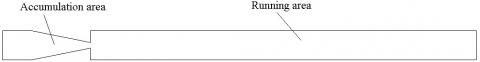(a) Plan of the model

## 1b.jpg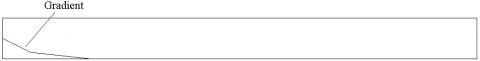(b) Profile of the model

Figure 1. Sketch of the dam-break model

Table 1. Calculation for operating conditions

 Operating condition Viscosity Coefficient Heights Gradient Pa $\cdot s$ m ° 1 3.0 0.0 0.0 2 5.2 0.0 0.0 3 7.0 0.0 0.0 4 5.2 0.1 0.0 5 5.2 0.2 0.0 6 5.2 0.0 2.5 7 5.2 0.0 5.0
3. Mathematical Model

3.1 Governing equation

The continuity equation:

$\frac{\partial U_{i}}{\partial X_{i}}=0$  (1)

The momentum equation:

$\frac{\partial U_{i}}{\partial t}+U_{j} \frac{\partial U_{i}}{\partial X_{j}}=-\frac{1}{\rho} \frac{\partial P}{\partial X_{i}}+\frac{\partial}{\partial X_{j}}\left(v \frac{\partial U_{i}}{\partial X_{j}}-\overline{u_{i} u_{j}}\right)+\frac{1}{\rho} F_{i}$   (2)

The equation $k$:

$\frac{\partial k}{\partial t}+U_{j} \frac{\partial k}{\partial X_{j}}=\frac{\partial}{\partial X_{j}}\left[\left(v+\frac{v_{t}}{\sigma_{k}}\right) \cdot \frac{\partial k}{\partial X_{j}}\right]+G-\varepsilon$     (3)

The equation $\varepsilon$

$\frac{\partial \varepsilon}{\partial t}+U_{j} \frac{\partial \varepsilon}{\partial X_{j}}=\frac{\partial}{\partial X_{j}}\left[\left(v+\frac{v_{t}}{\sigma_{\varepsilon}}\right) \cdot \frac{\partial \varepsilon}{\partial X_{j}}\right]+C_{1 \varepsilon} \frac{\varepsilon}{k} G-C_{2 \varepsilon} \frac{\varepsilon^{2}}{k}$     (4)

In the equations above,$-\overline{u_{i} u_{j}}=v_{t}\left(\frac{\partial U_{i}}{\partial X_{j}}+\frac{\partial U_{j}}{\partial X_{i}}\right)-\frac{2}{3} k \delta_{i j}$ , $\delta_{i j}$ is the symbol for Kronecker. When i=j, $\delta_{i j}$=1; When i≠j, $\delta_{i j}$=0. G is the generation of shear stress, and the expression is $G=v_{t}\left(\frac{\partial U_{i}}{\partial X_{j}}+\frac{\partial U_{j}}{\partial X_{i}}\right) \frac{\partial U_{i}}{\partial X_{j}}$; $\rho$ is the fluid density; p is the pressure;  t is the time; $U_{i}$ is the velocity component in $i$ direction;  $F_{i}$ is the body force acting on per unit of water;  $k=\overline{u_{i} u_{i}^{\prime}} / 2$ ,  is the turbulent kinetic energy per unit mass; ε is the turbulent dissipation rate;  ν is the kinematic coefficient of viscosity, which is given by Bingham equation; $v_{t}$ is the viscosity coefficient of turbulent motion, which is determined by the turbulent kinetic energy k and the turbulent dissipation rate ε;  $v_{t}=C_{\mu} \frac{k^{2}}{\varepsilon}$ . Cμ, C1ε, C2ε, σk and σε are the constants of the mode with respective valves of 0.09, 1.44, 1.92, 1.0 and 1.3.

Because it pertains to the hyper-concentration flow movement, the characteristics of debris flow conform to Bingham fluid [8, 9]. Hence the debris flow is studied through the Bingham model in this thesis. The kinematic coefficient of viscosity is given by the following Bingham equation:

$v=\frac{\mu}{\rho}=\left(K \gamma^{n-1}+\tau_{\mathrm{y}} / \gamma\right) / \rho$

In the equation,$\mu$ is the dynamic viscosity, and

$\gamma=\left[\frac{1}{2}(\Delta \cdot \Delta)\right]^{1 / 2}, \Delta=0.5\left(\frac{\partial u_{i}}{\partial x_{j}}+\frac{\partial u_{j}}{\partial x_{i}}\right)$

3.2 Grid division and boundary conditions

Rectangular grids are applied to the model in order to divide the computational domain in the study (the model grid shown in Figure 2). Each with a size of 0.01*0.01*0.01m, the number of grids is 1.26 million in total. The outflow boundary conditions are set in the outlet of the computational region, and the movement of debris flow is mainly determined by gravity.

## 2.png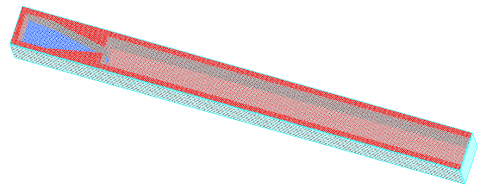Figure 2. Grid map of the computational model

4. Result and Analysis

4.1 The influence of dynamic viscosity on debris flow

The numerical simulations of three different dynamic viscosities (OC1, 2, 3) were carried out with the height of the dam and the gradient of the running area remaining unchanged. The results are shown in Table 2, Fig.3 and Fig.4. As the simulation results show, the dynamic viscosity has a significant impact on debris flow. When it is 3.0, the maximum moving distance of debris flow is 6.5m, and the maximum thickness is 0.046m; when it is 5.2, the maximum moving distance of debris flow is 5.0m, and the maximum thickness is 0.056m; when it is 7.0, the maximum moving distance of debris flow is 4.4m, and the maximum thickness is 0.063m. While the height of the dam and gradient remain unchanged, the higher the dynamic viscosity is, the shorter the moving distance is, and the larger the thickness is.

Table 2. Thickness for different dynamic viscosities

 Distance OC1 OC2 OC3 m m 0.0 0.046 0.056 0.063 0.6 0.045 0.056 0.062 1.0 0.045 0.055 0.059 2.0 0.043 0.052 0.055 3.0 0.041 0.047 0.047 4.0 0.036 0.038 0.033 4.4 0.033 0.031 0.000 4.5 0.032 0.029 5.0 0.028 0.000 6.0 0.021 6.5 0.000 7.0

## 3.png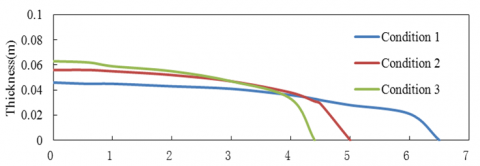Figure 3. Comparison of the moving distance of debris flow with the thickness

## 4a.png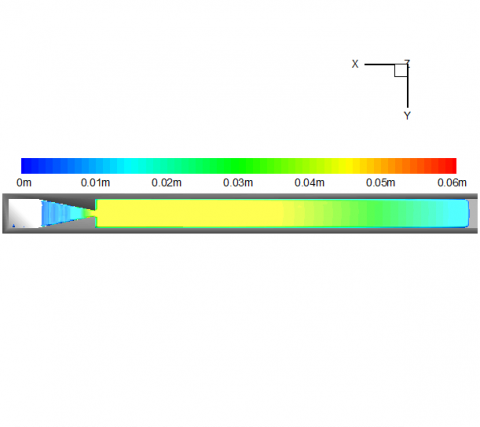(a) Dynamic viscosity=3.0 $P a \cdot s$

## 4b.png(b) Dynamic viscosity =5.2 $P a \cdot s$

## 4c.png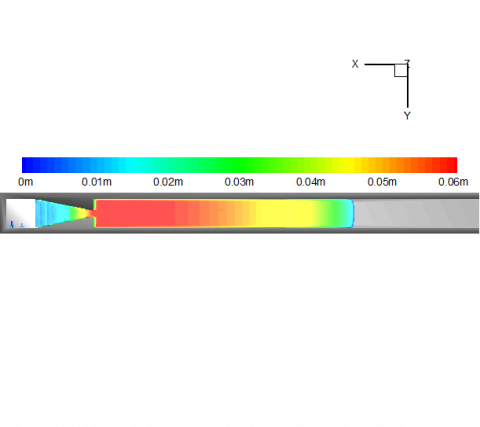(c) Dynamic viscosity =7.0 $P a \cdot s$

Figure 4. Plan for different dynamic viscosities of debris flow

4.2 The influence of dam height on debris flow

The numerical simulations of three different dam heights (OC2, 4, 5) were carried out with the dynamic viscosity and the gradient of the running area remaining unchanged. The results are shown in Table 3, Fig. 5 and Fig. 6. As the simulation results show, the dam height has an impact on debris flow. When it is 0.0m, the maximum moving distance of debris flow is 5.0m, and the maximum thickness is 0.056m; when it is 0.1m, the maximum moving distance of debris flow is 5.1m, and the maximum thickness is 0.055m; when it is 0.2, the maximum moving distance of debris flow is 5.2m, and the maximum thickness is 0.055m. While the dynamic viscosity and gradient remain unchanged, the higher the height of dam becomes, the longer the moving distance is, and the thickness remains fairly static.

Table 3. Thickness for different dam heights

 Distance OC 2 OC 4 OC 5 m m 0.0 0.056 0.052 0.050 0.6 0.056 0.055 0.055 1.0 0.055 0.055 0.055 2.0 0.052 0.052 0.052 3.0 0.047 0.047 0.047 4.0 0.038 0.04 0.04 5.0 0.000 0.016 0.022 5.1 0.000 0.014 5.2 0.000 6.0

## 5.png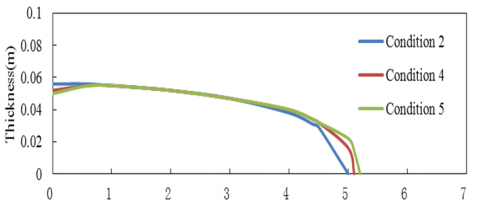Figure 5. Comparison of the moving distance of debris flow with the thickness

## 6a.png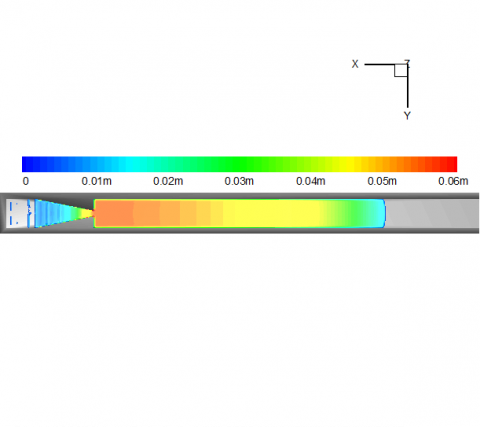(a) Dam height=0.0m

## 6b.png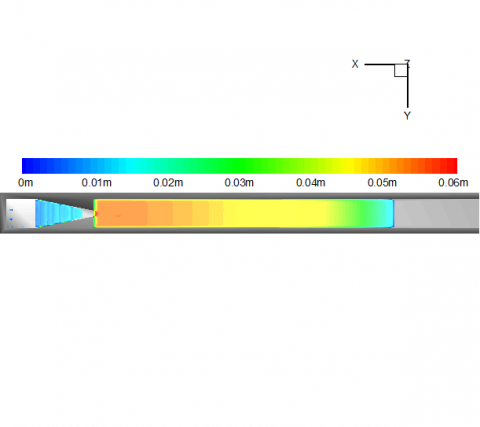(b) Dam height=0.1m

## 6c.png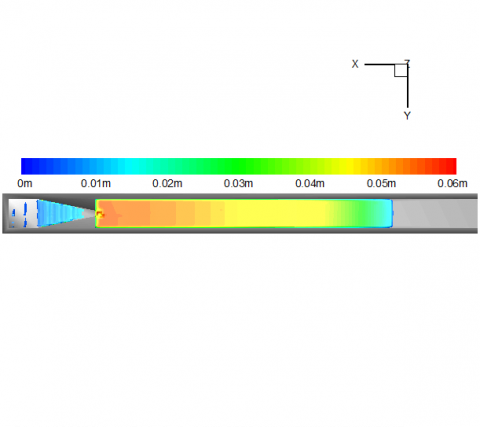(c) Dam height=0.2m

Figure 6. Plan for different dam heights of debris flow

4.3 The influence of dam height on debris flow

The numerical simulations of three different gradients of running area (OC2, 6, 7) were carried out with the dynamic viscosity and the gradient remaining unchanged. The results are shown in Table 4, Fig.7 and Fig.8. As the simulation results show, the gradient of the running area has a great impact on debris flow. When it is 0°, the maximum moving distance of debris flow is 5.0m, and the maximum thickness is 0.056m; when it is 2.5°, the maximum moving distance of debris flow is 5.4m, and the maximum thickness is 0.050m; when it is 5°, the maximum moving distance of debris flow is 6.2m, and the maximum thickness is 0.041m. While the dynamic viscosity and the dam height remain unchanged, the larger the gradient of the running area becomes, the longer the moving distance is, and the smaller the thickness is.

Table 4. Thickness for different gradients

 Distance OC 2 OC 6 OC 7 m m 0.0 0.056 0.042 0.032 0.6 0.056 0.045 0.034 1.0 0.055 0.048 0.036 2.0 0.052 0.049 0.039 3.0 0.047 0.050 0.040 4.0 0.038 0.044 0.041 5.0 0.000 0.031 0.041 5.4 0.000 0.037 6.0 0.025 6.2 0.000 7.0

## 7.pngFigure 7. Comparison of the moving distance of debris flow with the thickness

## 8a.png## 8b.png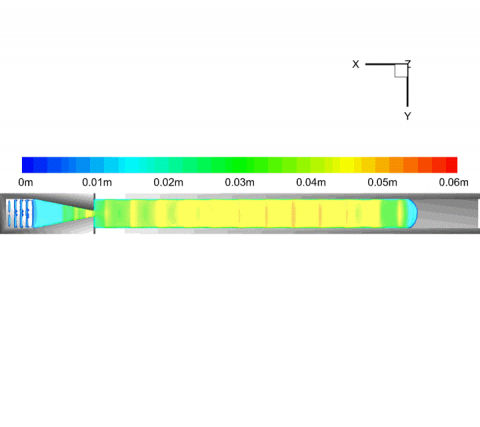## 8c.pngFigure 8. Plan for different running area gradients of debris flow

5. Conclusion

Taking the indoor dam-break model as an example, this thesis adopts a 3D numerical model to simulate the debris flow movement under various dynamics viscosities, heights of dam break, and gradients of running area. The influences of the above parameters on debris flow were analyzed by comparing the data of various operating conditions. The main results are as follows.

Firstly, by changing the dynamic viscosity, three different dynamic viscosities for debris flow were numerically simulated.  By comparing three operating conditions, it has been determined that the dynamic viscosity has a significant effect on the movement of debris flow. With the increase of the dynamic viscosity, the moving distance of debris flow decreases, and the maximum thickness increases.

Secondly, by changing the height of the bottom slope in the accumulation area, three different dam heights for debris flow were numerically simulated. By comparing three different operating conditions, it has been determined that the dam height has an effect on the movement of debris flow. With the increase of the dam height, the moving distance of debris flow increases, but only slightly, and the maximum thickness remain fairly static.

Thirdly, by changing the gradient, three different gradients in the running area of the debris flow were numerically simulated. Through the comparison of three operating conditions, it has been determined that the dynamic viscosity has a significant effect on the movement of the debris flow. With the increase of the gradient in the running area, the moving distance of the debris flow increases, and the maximum thickness decreases.

References

1. Q. L. Qi, L. T. Zhang, Numerical Simulation and Application of Seepage Field of Tailing Pond, Beijing: China Water & Power Press, 2011.

2. S. J. Ma et al., Release of Heavy Metal Ion Copper and Lead of Molybdenum Mine Tailings, Journal of China University of Mining & Technology, vol. 38, pp. 829-

834, 2009.

3. W. Q. Tian, J. G. Xue, Safety Technology and Management of Tailings Pond, Beijing: China Coal Industry Publishing House, 2006.

4. B. Yuan et al., Study on the Model for Tailing Dam Breaking and Its Application, China Safety Science Journal, vol. 18, pp. 169-172, 2008.

5. G. Z. Yin et al., Experimental Study of Similar Simulation of Tailings Dam-Break, Chinese Journal of Rock Mechanics and Engineering, vol. 18, pp. 169-172, 2008.

6. X. F. Jing et al., Model Experimental Study of Collapse Mechanism and Broken Mode of Tailings Dam, Rock and Soil Mechanics, vol. 29, pp. 3830-3838, 2010.

7. L. T. Zhang, Summary on the Dam-Break of Tailing Pond, Journal of Hydraulic Engineering, vol. 44, pp. 594-600, 2013.

8. Ai-Hamad M., Duwairi H. M. Effect of Heat Generation/Absorption on Heat Transfer for a Non-Newtonian Fluid, International Journal of Heat and Technology, vol. 26, pp. 115-120, 2013.

9. Coppi M., Quintino A., Salata F., Fluid Dynamic Feasibility Study of Solar Chimney in Residentail Buildings, International Journal of Heat and Technology, vol. 29, pp. 1-5, 2011.

10. L. T. Zhang et al., 3D Numerical Simulation on Evolution of Debris Flow from Dam Break of Tailing Pond, International Journal of Earth Sciences and Engineering, vol. 7, pp. 111-115, 2014.

11. A. Caliskan, S. Elci, Effects of Selective Withdrawal on Hydrodynamics of a Stratified reservoir, Water Resour Manage, vol. 23, pp. 1257-1273. DOI: 10.1007/s11269-008-9325-x.

12. J. M. Hamrick. Users’ Manual for Environmental Fluid Dynamics Computer Code, Virginia Insitute of Marine Science, 1996.

13. S. W. Ma et al., Effects of Selective Water Withdrawal Schemes on Thermal Stratification in Kouris Dam in Cyprus, Lakes & Reservoirs: Research and Management, vol. 13, pp. 51-61, 2008. DOI: 10.1111/j.1440-1770.2007.00353.x.

14. B. Tartinville, E. Deleersnijder, J. Rancher, The Water Residence Time in the Mururoa Atoll Lagoon: Sensitivity Analysis of a Three-Dimensional Model, Coral Reefs, vol. 17, pp. 193-203, 1997. DOI: 10.1007/s003380050074.